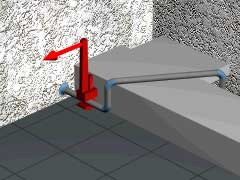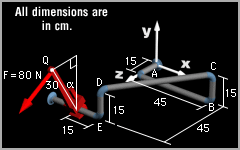Ch 4. Moments/Equivalent Systems Multimedia Engineering Statics Moment2-D Scalar Moment3-D Scalar Moment3-D Vector Couples and Equiv. System Distributed Loads, Intro
 Chapter 1. Basics 2. Vectors 3. Forces 4. Moments 5. Rigid Bodies 6. Structures 7. Centroids/Inertia 8. Internal Loads 9. Friction 10. Work & Energy Appendix Basic Math Units Sections Search eBooks Dynamics Fluids Math Mechanics Statics Thermodynamics Author(s): Kurt Gramoll ©Kurt GramollSTATICS - CASE STUDY IntroductionProblem Graphic In the previous section, the moment generated by a wrench as analyzed. However, it was determined that the moment changed as the wrench was rotated around the pipe. It is possible that the magnitude of the moment will increase even though the magnitude of the force remains constant. What is known: The wrench rotates from α = 0° to α = 90°. The force is applied at point Q. The necessary dimensions are given. QuestionsKnown information What is the maximum moment generated at joint B as the wrench rotates from α = 0° to α = 90°? Approach Determine the position vector from joint B to point Q for any angle α. Determine the Cartesian components of F as a function of the angle α. Solve for the moment of F at joint B using the vector description of moment.

Practice Homework and Test problems now available in the 'Eng Statics' mobile app
Includes over 500 problems with complete detailed solutions.
Available now at the Google Play Store and Apple App Store.